# Olympiad Test : Ratio And Proportion - 1

## 10 Questions MCQ Test Maths Olympiad Class 6 | Olympiad Test : Ratio And Proportion - 1

Description
Attempt Olympiad Test : Ratio And Proportion - 1 | 10 questions in 20 minutes | Mock test for Class 6 preparation | Free important questions MCQ to study Maths Olympiad Class 6 for Class 6 Exam | Download free PDF with solutions
QUESTION: 1

### 12 boys can dig a pitch in 12 hours. How long will 18 boys take to do it ?

Solution:

12 boys can dig a pitch in 12 hours
∴ 1 boy can dig a pitch in 12 × 12 hours
Hence, 18 boys can dig a pitch in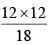= 8 hours

QUESTION: 2

### If a bus covers 225 km in 3 hours and a train covers 600 km in 5 hours. What is the ratio of their speeds?

Solution: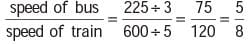QUESTION: 3

### The angles of a triangle are in the ratio 2 : 3 : 5. What is the measure of the largest angle?

Solution:

Here, 2x + 3x + 5x = 180
⇒ 10x = 180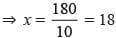∴ Largest angle = 5x = 5 × 18 = 90°

QUESTION: 4

If 36 men can finish a piece of work in 36 days. How many men will be required to finish it in 24 days?

Solution:

In 36 days the work is finished by 36 men
In 1 day the work is finished 36 × 36
On 24 days the work is finished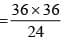= 54 men

QUESTION: 5

If x, y, z are in proportion then which of the following is true?

Solution:

If x, y, z are in proportion then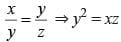QUESTION: 6

If 35 envelop costs Rs 87.50 how many such envelops can we purchase for Rs 315?

Solution:

No. of envelops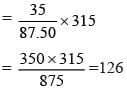QUESTION: 7

The weight of 65 magzines is 13 kg. What is the weight of 80 such magazines?

Solution:

Weight of 65 magazines = 13 kg
∴ Weight of 1 magazine = 13/65 kg
Weight of 80 magazines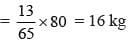QUESTION: 8

The boys and girls in a school are in the ratio 9 : 5. If the total strength of the school is 994. What is the number of girls?

Solution:

Given,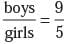∴ No. of girls =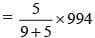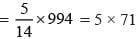= 355

QUESTION: 9

The ratio of length of a field to its width is 5 : 3. What is the length if the width is 36 cm?

Solution:

Given,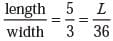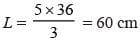QUESTION: 10

If Rs 1020 is divided among A, B, C in the ratio 2 : 3 : 5. What is the share of C?

Solution:

Given,
2x + 3x + 5x = 1020
⇒ 10x = 1020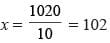∴ Share of C = 5 × 102 = 510Use Code STAYHOME200 and get INR 200 additional OFF Use Coupon Code